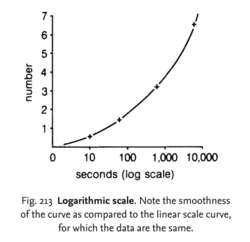# logarithmic scale

(redirected from Logarithmic graph paper)
Also found in: Dictionary, Thesaurus, Financial, Encyclopedia.Fig. 213 Logarithmic scale . Note the smoothness of the curve as compared to the linear scale curve, for which the data are the same.

## logarithmic scale

a scale in which the values of a variable are expressed as logarithms. Such transformations of data are often employed to simplify the drawing of lines on a graph, where the variable values are spread over a wide range. See Fig. 213 . A good example of the use of log scales is seen in Fig. 182 where the LOG PHASE of a microbial GROWTH CURVE is illustrated. Compare LINEAR SCALE.
Collins Dictionary of Biology, 3rd ed. © W. G. Hale, V. A. Saunders, J. P. Margham 2005
Site: Follow: Share:
Open / Close